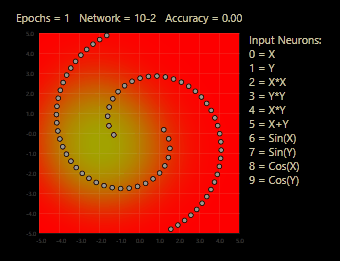## A Comparison of Symmetric Kullback-Leibler, Jensen-Shannon, and Hellinger Distances

A surprisingly common task in machine learning is to compute the distance between (or similarity to) two probability or frequency distributions. For example, suppose P = (0.20, 0.50, 0.30) and Q = (0.10, 0.30, 0.60). What is the distance between P and Q?

There are many ways to compute a distance. Three of the most common are Kullback-Leibler (KL), symmetric Kullback-Leibler (sKL), Jensen-Shannon (JS), and Hellinger (H).

Note: Another common distance is the Wasserstein distance, also known as the Earth Mover distance, but it’s more difficult to compute so I don’t compare it with KL, JS, H. Theoretically, Wasserstein is supposed to be superior to KL, JS, and H distances. I intend to figure out how to implement Wasserstein distance from scratch, rather than using the scipy library wasserstein_distance() function, and then I’ll compare Wasserstein distance with KL, JS, and H distances.Four probability distributions for my investigations.After I learned how to compute these distances, the next question was, “Which one is best under which circumstances?” There is no good information available on the Internet. But here are my observations.

Regular KL is not good because KL(P,Q) != KL(Q,P) — it’s not symmetric. There are several ways to make a symmetric KL. The simplest is to define sKL(P,Q) = KL(P,Q) + KL(Q,P) — just sum both directions. However, none of the simple symmetric versions of KL satisfy the triangle inequality (TI).Top: equation for Kullback-Leibler distance. Middle: Jensen-Shannon. Bottom: Hellinger.

For a distance function D and distributions P, Q, R, if D(P,R) <= D(P,Q) + D(P,R) then the distance function D satisfies the triangle inequality, which is a nice characteristic.

The Jensen-Shannon and Hellinger distance functions satisfy three nice properties that qualify them as formal “metrics” (as opposed to the general meaning a of a number that measures something).

1. D(P,Q) >= 0 and D(P,Q) == 0 IFF P == Q
2. D(P,Q) == D(Q,P)
3. D(P,R) <= D(P,Q) + D(Q,R)

I wrote a short demo program to look at sKL, JS, and H distance and a few sample distributions. I was surprised that JS and H gave very similar results. The fact that KL does not satisfy the triangle inequality is a negative feature, so, in short, I judge JS and H distance preferable to KL and symmetric KL for most scenarios.

From an engineering perspective, JS(P,Q) has a minor downside that a naive computation will fail if you have paired values that sum to 0.0 — for example, P = [0.20, 0.00, 0.80] and Q = [0.40, 0.00, 0.60] will invoke a log(0.0) which is negative infinity. Hellinger distance doesn't have this kind of minor engineering glitch to watch-for.

Ultimately, if one distance function was clearly best, there would only be one distance function. The fact that there are so many distance functions suggests that either 1.) all work pretty much the same, or 2.) different functions work best for different problems, or 3.) both.

So, there is no definitive answer to, “Which distance function is best?” If you have a choice, Hellinger distance is appealing, but Wasserstein distance might be better (TBD based on some future experiments).The artist who identifies himself as “batjorge” describes himself as an “iterative fractalist”. Whoever or whatever he is, he creates beautiful digital images where the distance between reality and art is just enough to make the images appealing to me.

This entry was posted in Machine Learning. Bookmark the permalink.

### 3 Responses to A Comparison of Symmetric Kullback-Leibler, Jensen-Shannon, and Hellinger Distances

1.Thorsten Kleppe says:

Very nice post.

•jamesdmccaffrey says:

Thank you Thorsten. I hope you’re doing well! JM

•Thorsten Kleppe says:

Hi James, so far all is well, I hope for you too.
Right now I’m doing feature engineering. I have created a little demo, isn’t that crazy?The Tensorflow Playground example with all features and the circle dataset gives an idea how this can be possible without hidden layers. I called it curved linearity, with a little more features it can actually solve simple non-linear problems. All attempts only because of mixed linearity, this cool feature I described in custom connect:

https://github.com/grensen/custom_connect